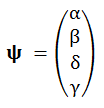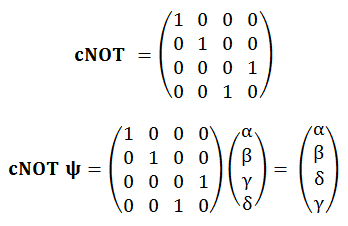## Sunday, 23 October 2011

### Quantum Computing #2: Multiple Qubit Gates

Previously:
Introduction
Single Qubit Gates.
Entanglement

Last time we saw that a single qubit can be in either basis state, 0 or 1, or any normalized superposition of the two:

ψ = α0 + β1, where |α|² + |β|² = 1.

When we come to analyse systems of two or more qubits, it's important to realise that we don't just replicate the above, once per additional qubit, as we would in classical logic. This is because qubit states can be entangled. They have to be treated as a whole: as the overall state of the qubit set. Therefore, to take the next simplest example, a two-qubit system can be in any of the four basis states, 00, 01, 10 or 11, as well as any normalized superposition of these four:

ψ = α00 + β01 + γ10 + δ11, where |α|² + |β|² + |γ|² + |δ|² = 1.

As before, we can introduce an arbitrary set of orthogonal column vectors to represent each of these four basis states,which gives us this representation of the multi-qubit state as a column vector:and in general, an n-qubit system will have 2ⁿ pairwise independent (orthogonal) states in its computational basis, and hence 2ⁿ components in its state column vector.

The Controlled-NOT Gate

Recall what single-qubit gates such as X and H looked like: 2x2 unitary matrices operating on unit state column vectors. Similarly, n-qubit gates are represented by unitary 2ⁿx2ⁿ matrices. Our next example is termed the Controlled-NOT gate, or cNOT (feel free to invent your own pronunciation). This has two inputs and two outputs. The first input is passed through unchanged to the corresponding output. When this first input is 0, the second input is also passed through unchanged to its corresponding output. But when the first input is 1, the second input gets inverted as through an X gate. Here is the matrix representation of cNOT, and its operation upon a general 2-qubit state column vector:To see exactly how this matrix represents a cNOT operation on two qubits, we consider its effect upon each of the four basis states in turn:By checking each of these four cases in turn, we can confirm that the gate operates as advertised in the description above. Finally for the sake of completeness, and courtesy of Wikipedia, here is the circuit diagram symbol of a cNOT gate. The top quantum wire represents the "control" qubit, which is transmitted unaltered, while the bottom path represents the "controlled", i.e. conditionally inverted, qubit.Miscellany

That's the meat of the present article in the series. I'll finish with a couple of general remarks on what we've seen so far.

There's actually an entire family of controlled gates similar to cNOT, differing only in the particular single-qubit operation that's applied to the controlled qubit when the control is 1. Their matrices are easily obtained by substituting the single-qubit operation's 2x2 sub matrix in the bottom right quadrant of cNOT.

All quantum gates have square matrix representations, since they all have the same number of outputs as inputs. That's because quantum computations have to be reversible. It's easy to show that gates like the classical AND, OR etc. can't be made reversible, as there's less information in their output (one bit) than in their inputs (two or more bits). This also implies that those classical gates necessarily dissipate energy during their operation.

Next time: Measurement; also, a Review of the story so far, devoid of matrices & Greek letters!